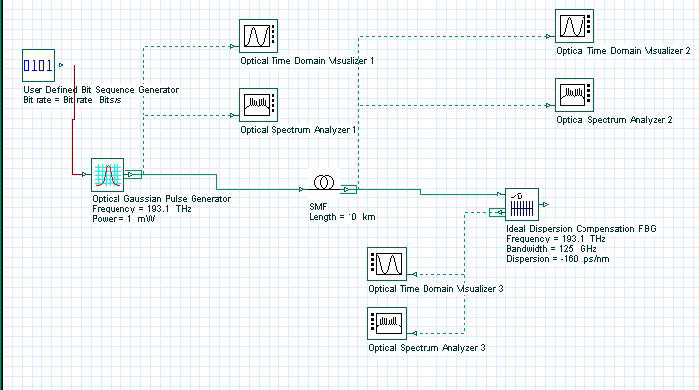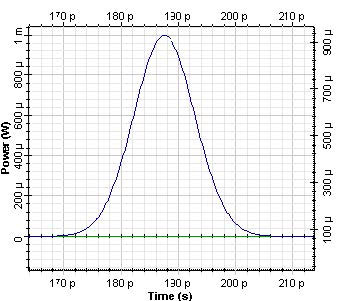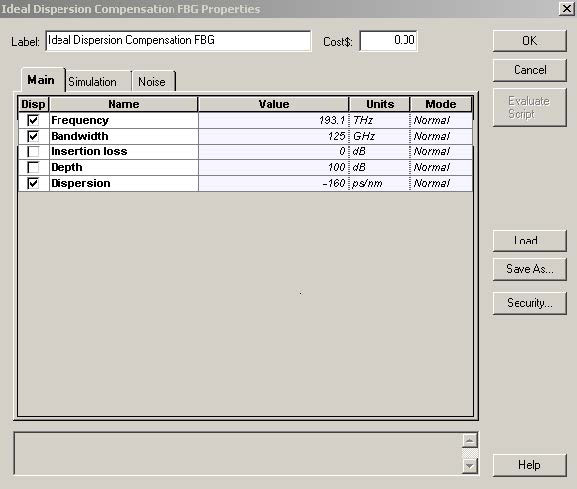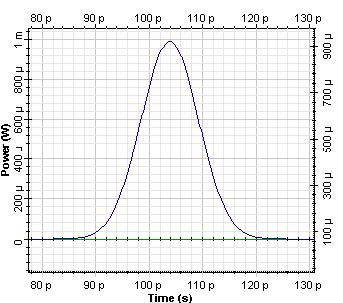This lesson demonstrates the possibility for dispersion compensation with the help of ideal dispersion component in OptiSystem.

The project layout is shown in Figure 1.Figure 1: Project Layout for dispersion compensation with ideal dispersion component in OptiSystem

The following shape was generated in the Optical Gaussian pulse generator with an initial 12.5 ps pulse and global parameters of a 40 Gb/s bit rate and a 0.5 times bit slot.Figure 2: Initial gaussian pulse

The pulse was launched in 10 km SMF. As a result of this propagation, the width of the pulse increases approximately four times.Figure 3: Gaussian pulse after 10 km propagation in SMF

After 10 km propagation in SMF, the accumulated dispersion is 160 ps/nm. In order to compensate for this accumulated dispersion, the corresponding option Dispersion in the Main tab of the Ideal Dispersion Compensation component is fixed as – 160 ps/nm. The central frequency and corresponding bandwidth (in this case ~ 3 times the bit rate) has to be properly chosen. The corresponding tab with these parameters for this component is shown in Figure 4.Figure 4: Parameters of Ideal Dispersion Compensation component

The result of dispersion compensation performed with the Ideal Dispersion Compensation component is shown in Figure 5.Figure 5: Pulse after dispersion compensation with Ideal Dispersion Compensation component

As we could expect, an exact compensation of accumulated dispersion was achieved.

In conclusion, we have shown in this session how to use an Ideal Dispersion Compensation component in OptiSystem for dispersion compensation.